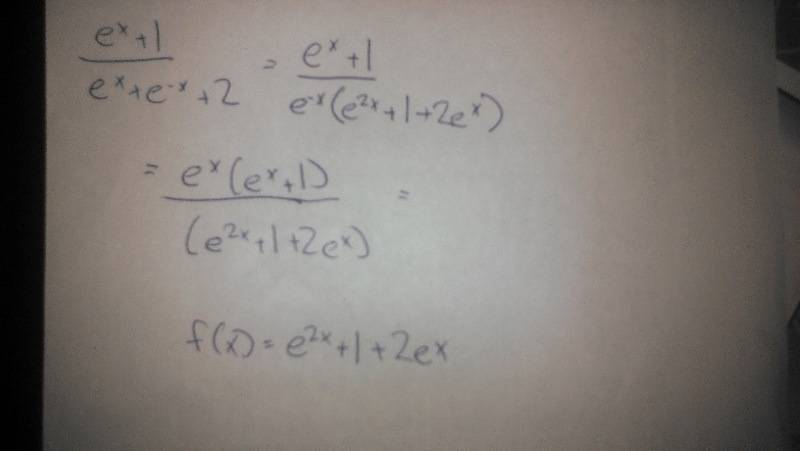# Integration using a specific method

Charlotte87

## Homework Statement

I am suppose to rearrange the integral in order to use this formula to solve it:
∫f'(x)/f(x)dx=ln|f(x)|+C

## Homework Equations

My integral is ∫(e^x+1)/(e^x+e^-x+2).

## The Attempt at a Solution

Any help?

Gold Member
To simplify the integral, let ##y=e^x## and break it into partial fractions.

Last edited:
Saitama
Write e^(-x) as 1/(e^x). Simplify and you should get the expression:
$$\frac{e^{2x}+e^x}{e^{2x}+2e^x+1}$$
Can you now see which is f(x) and which is f'(x)?

smk037
this is an easy way to do it

should get you started at leastsmk037
pranav got there before me... lol